Analytic Functions

Analytic functions compute an aggregate value based on a group of rows. They differ from aggregate functions in that they return multiple rows for each group. The group of rows is called a window and is defined by the analytic_clause. For each row, a sliding window of rows is defined. The window determines the range of rows used to perform the calculations for the current row. Window sizes can be based on either a physical number of rows or a logical interval such as time.

Analytic functions are the last set of operations performed in a query except for the final ORDER BY clause. All joins and all WHERE, GROUP BY, and HAVING clauses are completed before the analytic functions are processed. Therefore, analytic functions can appear only in the select list or ORDER BY clause.

Analytic functions are commonly used to compute cumulative, moving, centered, and reporting aggregates.

analytic_function::=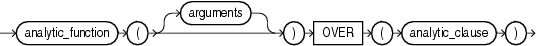Description of the illustration analytic_function.gif

analytic_clause::=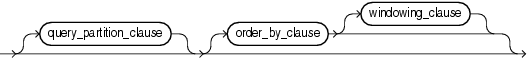Description of the illustration analytic_clause.gif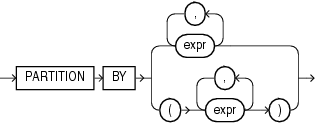Description of the illustration query_partition_clause.gif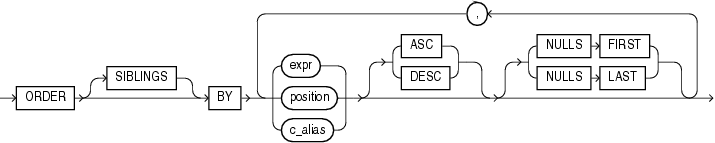Description of the illustration order_by_clause.gif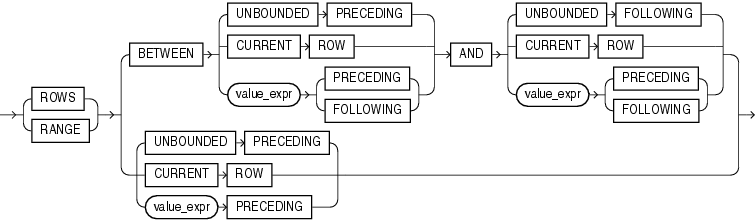Description of the illustration windowing_clause.gif

The semantics of this syntax are discussed in the sections that follow.

analytic_function

Specify the name of an analytic function (see the listing of analytic functions following this discussion of semantics).

arguments

Analytic functions take 0 to 3 arguments. The arguments can be any numeric data type or any nonnumeric data type that can be implicitly converted to a numeric data type. Oracle determines the argument with the highest numeric precedence and implicitly converts the remaining arguments to that data type. The return type is also that data type, unless otherwise noted for an individual function.

"Numeric Precedence" for information on numeric precedence and Table 3-10, "Implicit Type Conversion Matrix" for more information on implicit conversion

analytic_clause

Use OVER analytic_clause to indicate that the function operates on a query result set. This clause is computed after the FROM, WHERE, GROUP BY, and HAVING clauses. You can specify analytic functions with this clause in the select list or ORDER BY clause. To filter the results of a query based on an analytic function, nest these functions within the parent query, and then filter the results of the nested subquery.

Notes on the analytic_clauseThe following notes apply to the analytic_clause:

• You cannot nest analytic functions by specifying any analytic function in any part of the analytic_clause. However, you can specify an analytic function in a subquery and compute another analytic function over it.

• You can specify OVER analytic_clause with user-defined analytic functions as well as built-in analytic functions. See CREATE FUNCTION.

query_partition_clause

Use the PARTITION BY clause to partition the query result set into groups based on one or more value_expr. If you omit this clause, then the function treats all rows of the query result set as a single group.

To use the query_partition_clause in an analytic function, use the upper branch of the syntax (without parentheses). To use this clause in a model query (in the model_column_clauses) or a partitioned outer join (in the outer_join_clause), use the lower branch of the syntax (with parentheses).

You can specify multiple analytic functions in the same query, each with the same or different PARTITION BY keys.

If the objects being queried have the parallel attribute, and if you specify an analytic function with the query_partition_clause, then the function computations are parallelized as well.

Valid values of value_expr are constants, columns, nonanalytic functions, function expressions, or expressions involving any of these.

order_by_clause

Use the order_by_clause to specify how data is ordered within a partition. For all analytic functions you can order the values in a partition on multiple keys, each defined by a value_expr and each qualified by an ordering sequence.

Within each function, you can specify multiple ordering expressions. Doing so is especially useful when using functions that rank values, because the second expression can resolve ties between identical values for the first expression.

Whenever the order_by_clause results in identical values for multiple rows, the function behaves as follows:

• CUME_DIST, DENSE_RANK, NTILE, PERCENT_RANK, and RANK return the same result for each of the rows.

• ROW_NUMBER assigns each row a distinct value even if there is a tie based on the order_by_clause. The value is based on the order in which the row is processed, which may be nondeterministic if the ORDER BY does not guarantee a total ordering.

• For all other analytic functions, the result depends on the window specification. If you specify a logical window with the RANGE keyword, then the function returns the same result for each of the rows. If you specify a physical window with the ROWS keyword, then the result is nondeterministic.

Restrictions on the ORDER BY Clause The following restrictions apply to the ORDER BY clause:

• When used in an analytic function, the order_by_clause must take an expression (expr). The SIBLINGS keyword is not valid (it is relevant only in hierarchical queries). Position (position) and column aliases (c_alias) are also invalid. Otherwise this order_by_clause is the same as that used to order the overall query or subquery.

• An analytic function that uses the RANGE keyword can use multiple sort keys in its ORDER BY clause if it specifies any of the following windows:

• RANGE BETWEEN UNBOUNDED PRECEDING AND CURRENT ROW. The short form of this is RANGE UNBOUNDED PRECEDING.

• RANGE BETWEEN CURRENT ROW AND UNBOUNDED FOLLOWING

• RANGE BETWEEN CURRENT ROW AND CURRENT ROW

• RANGE BETWEEN UNBOUNDED PRECEDING AND UNBOUNDED FOLLOWING

Window boundaries other than these two can have only one sort key in the ORDER BY clause of the analytic function. This restriction does not apply to window boundaries specified by the ROW keyword.

ASC | DESC Specify the ordering sequence (ascending or descending). ASC is the default.

NULLS FIRST | NULLS LAST Specify whether returned rows containing nulls should appear first or last in the ordering sequence.

NULLS LAST is the default for ascending order, and NULLS FIRST is the default for descending order.

Analytic functions always operate on rows in the order specified in the order_by_clause of the function. However, the order_by_clause of the function does not guarantee the order of the result. Use the order_by_clause of the query to guarantee the final result ordering.

windowing_clause

Some analytic functions allow the windowing_clause. In the listing of analytic functions at the end of this section, the functions that allow the windowing_clause are followed by an asterisk (*).

ROWS | RANGE These keywords define for each row a window (a physical or logical set of rows) used for calculating the function result. The function is then applied to all the rows in the window. The window moves through the query result set or partition from top to bottom.

• ROWS specifies the window in physical units (rows).

• RANGE specifies the window as a logical offset.

You cannot specify this clause unless you have specified the order_by_clause. Some window boundaries defined by the RANGE clause let you specify only one expression in the order_by_clause. Refer to "Restrictions on the ORDER BY Clause".

The value returned by an analytic function with a logical offset is always deterministic. However, the value returned by an analytic function with a physical offset may produce nondeterministic results unless the ordering expression results in a unique ordering. You may have to specify multiple columns in the order_by_clause to achieve this unique ordering.

BETWEEN ... AND Use the BETWEEN ... AND clause to specify a start point and end point for the window. The first expression (before AND) defines the start point and the second expression (after AND) defines the end point.

If you omit BETWEEN and specify only one end point, then Oracle considers it the start point, and the end point defaults to the current row.

UNBOUNDED PRECEDING Specify UNBOUNDED PRECEDING to indicate that the window starts at the first row of the partition. This is the start point specification and cannot be used as an end point specification.

UNBOUNDED FOLLOWING Specify UNBOUNDED FOLLOWING to indicate that the window ends at the last row of the partition. This is the end point specification and cannot be used as a start point specification.

CURRENT ROW As a start point, CURRENT ROW specifies that the window begins at the current row or value (depending on whether you have specified ROW or RANGE, respectively). In this case the end point cannot be value_expr PRECEDING.

As an end point, CURRENT ROW specifies that the window ends at the current row or value (depending on whether you have specified ROW or RANGE, respectively). In this case the start point cannot be value_expr FOLLOWING.

value_expr PRECEDING or value_expr FOLLOWING For RANGE or ROW:

• If value_expr FOLLOWING is the start point, then the end point must be value_expr FOLLOWING.

• If value_expr PRECEDING is the end point, then the start point must be value_expr PRECEDING.

If you are defining a logical window defined by an interval of time in numeric format, then you may need to use conversion functions.

NUMTOYMINTERVAL and NUMTODSINTERVAL for information on converting numeric times into intervals

If you specified ROWS:

• value_expr is a physical offset. It must be a constant or expression and must evaluate to a positive numeric value.

• If value_expr is part of the start point, then it must evaluate to a row before the end point.

If you specified RANGE:

• value_expr is a logical offset. It must be a constant or expression that evaluates to a positive numeric value or an interval literal. Refer to "Literals" for information on interval literals.

• You can specify only one expression in the order_by_clause.

• If value_expr evaluates to a numeric value, then the ORDER BY expr must be a numeric or DATE data type.

• If value_expr evaluates to an interval value, then the ORDER BY expr must be a DATE data type.

If you omit the windowing_clause entirely, then the default is RANGE BETWEEN UNBOUNDED PRECEDING AND CURRENT ROW.

Analytic functions are commonly used in data warehousing environments. In the list of analytic functions that follows, functions followed by an asterisk (*) allow the full syntax, including the windowing_clause.

AVG *
CORR *
COUNT *
COVAR_POP *
COVAR_SAMP *
CUME_DIST
DENSE_RANK
FIRST
FIRST_VALUE *
LAG
LAST
LAST_VALUE *
LISTAGG
MAX *
MIN *
NTH_VALUE*
NTILE
PERCENT_RANK
PERCENTILE_CONT
PERCENTILE_DISC
RANK
RATIO_TO_REPORT
REGR_ (Linear Regression) Functions *
ROW_NUMBER
STDDEV *
STDDEV_POP *
STDDEV_SAMP *
SUM *
VAR_POP *
VAR_SAMP *
VARIANCE *